# Where goes the Energy lost by the Beam?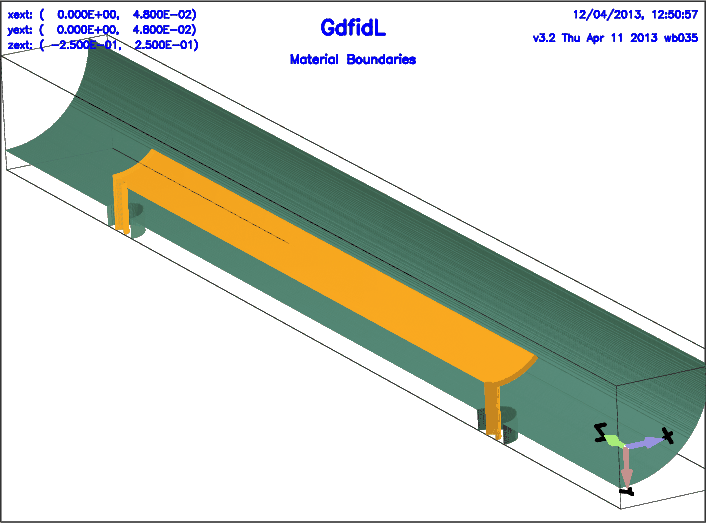Dr Alexei Blednykh of BNL had send us the Description of a Device where he wants to know where the Energy lost by the Beam goes. The Inputfile for this Device is Inputfile The Energy-Loss is the longitudinal Lossfactor. A Wakepotential Computation gives a Energy Loss of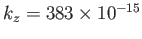Joules.

   gd1 < Inputfile | tee Logfile
echo "-gen, inf @last, -wakes, shigh= 0.1, doit" | gd1.pp


A Plot of the longitudinal Wakepotential is shown in Figure 2. The Lossfactor ofNewton-Meter is indicated in the upper right Corner. That Lossfactor as given by gd1.pp takes into Account the used two Planes of Symmetry.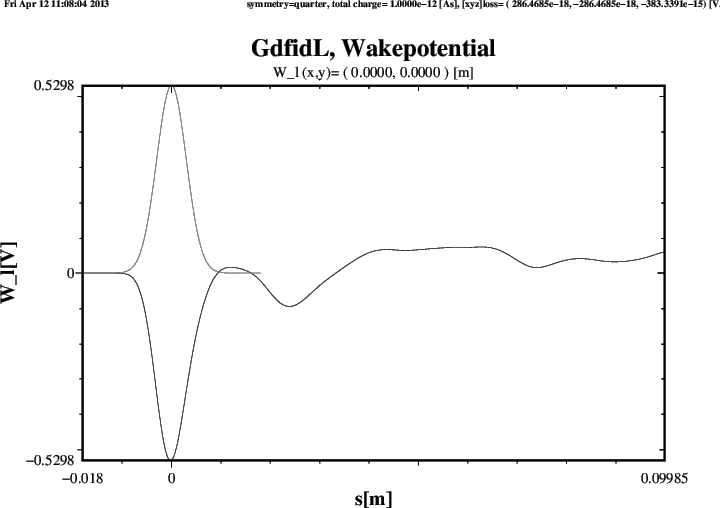The Energy in the considered Volume will leave through the Ports. We have a Look at the Signals at the Ports:

 # Input for gd1.pp:
-general, inf @last
-spara, freq no, time yes, upto 3e-9, doit

Three of the four resulting Plots are shown in Figures 3 through 5.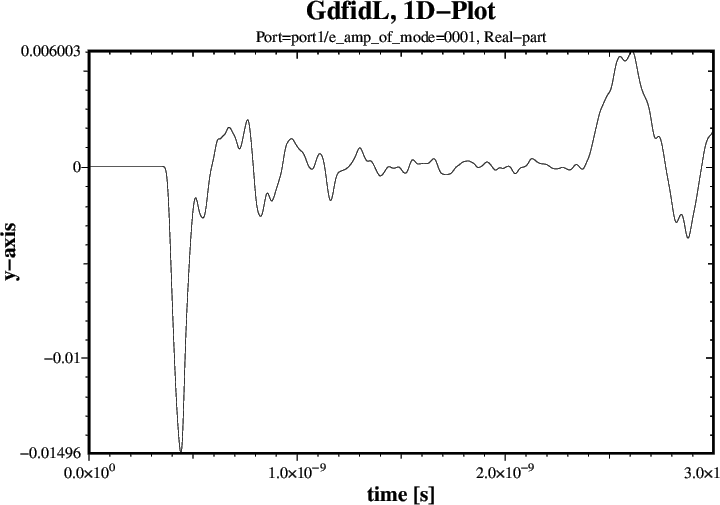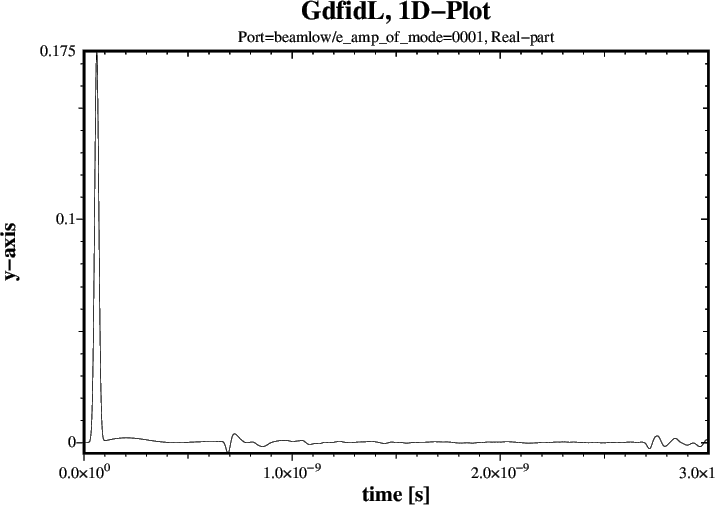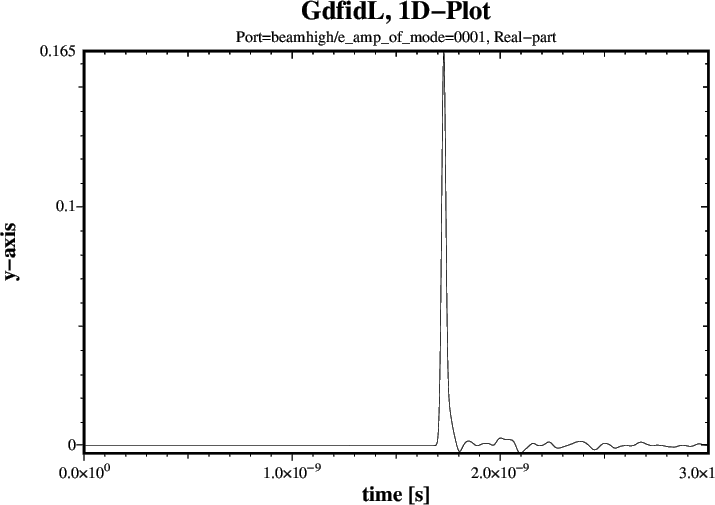We compute the integrated Power Sum of all Modes:

 # Input for gd1.pp:
-general, inf @last
-spara, freq no
time no,  # time= yes, would give us Plots of all Signals.
modes= all, ports= all
tintpower= yes
upto 3e-9
doit

The resulting Plot is shown in Figure 6. The reported Power up to t=3nS is 1.9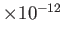, much larger than the Lossfactor. The Result is so much larger, as the Signals are partly corrupted by the Beam-Fields.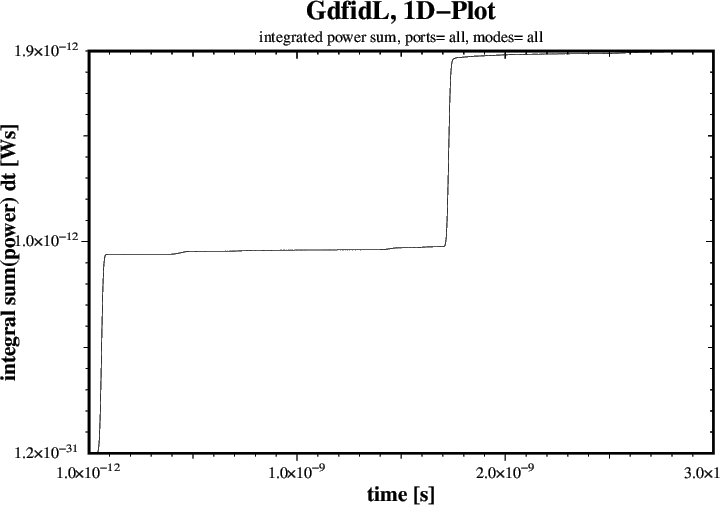We compute the Power in the Signals which are corrupted by the Beam Fields by chopping off the Parts which are corrupted.
 # Input for gd1.pp:
-general, inf @last
-spara, freq no
time no,  # time= yes, would give us Plots of all Signals.
tintpower= yes
upto 3e-9
modes= all,
ports= ( port1, port2), doit # These Signals are not corrupted.
tfirst= 0.4e-9, ports= ( beamlow ), doit # Corrupted up to t= 2*0.35e-9
tfirst= 1.76e-9, ports= ( beamhigh ), doit # Corrupted up to t= 1.76e-9

The resulting Plots are shown in Figures 7 through 9.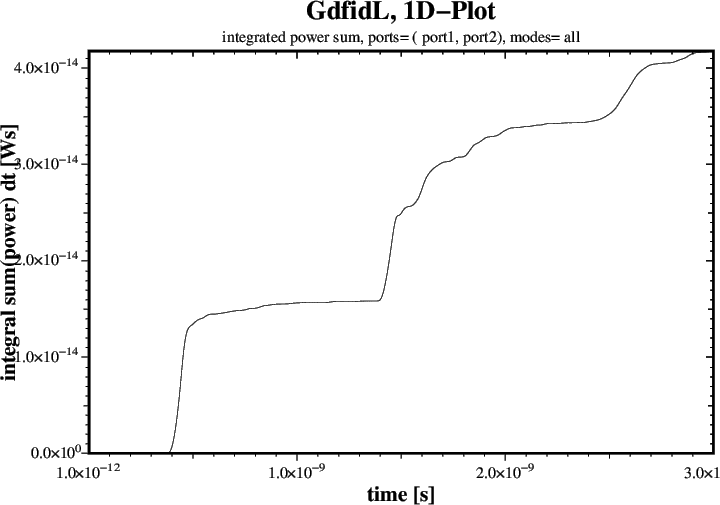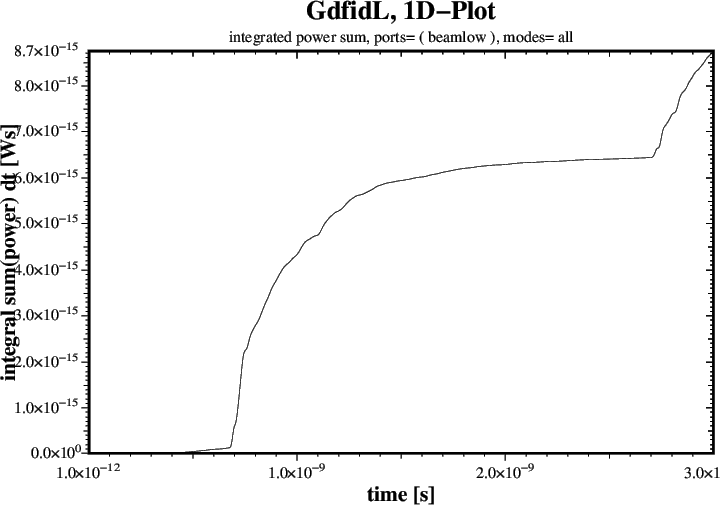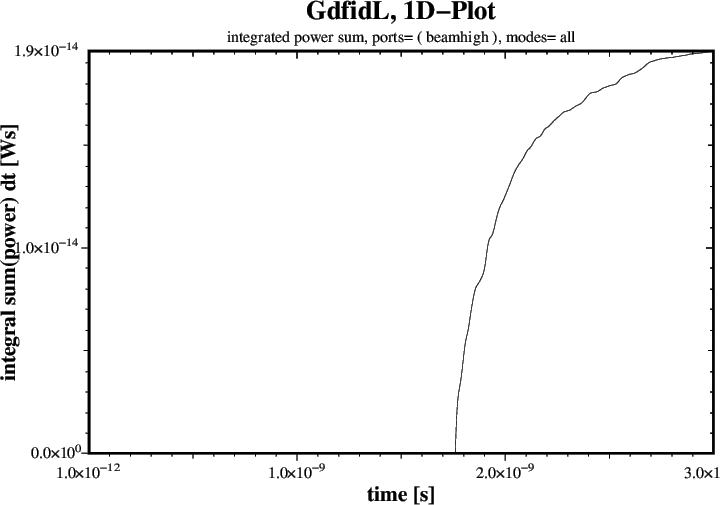What is the Energy which at t=3 nS has not yet left the Volume?
 # Input for gd1.pp:
-general, inf @last
-energy
symbol= e_2, doit
symbol= h_2, doit

This gives:
 ##############################################################################
# Flags: menu, prompt, message,                                              #
##############################################################################
# Section: -energy                                                           #
##############################################################################
# symbol   = h_2                                                             #
# quantity = h                                                               #
# solution = 2                                                               #
# bbxlow =    -1.0000e+30, bbylow =    -1.0000e+30, bbzlow =    -1.0000e+30  #
# bbxhigh=     1.0000e+30, bbyhigh=     1.0000e+30, bbzhigh=     1.0000e+30  #
#                                                                            #
#                                                                            #
# @henergy : 6.45617e-15               (symbol: h_2, m: 1)                   #
# @eenergy : 8.02293e-15               (symbol: e_2, m: 1)                   #
##############################################################################
# doit, ?, return, end, help, ls                                             #
##############################################################################

Our Energies so far:
     6.45617e-15    # H-Energy at t=3e-9
8.02293e-15    # E-Energy at t=3e-9
42e-15         # Energy left through Port1 and Port2
8.7e-15        # Energy left through BeamLow
19.5e-15       # Energy left through BeamHigh
Sum: 84.7e-15 Joules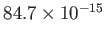Joules is much less than the Lossfactor of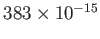Joules. We have to multiply theJoules by Four, as we used the two Planes of Symmetry. This then gives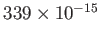Joules which have left the Volume or are still in the Volume. This is almost the Value of the Lossfactor. Where are the missing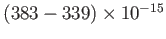Joules? They have left the Volume through the upper Port BeamHigh at Times when the Beam passed through the Port, and these Signals were chopped of.